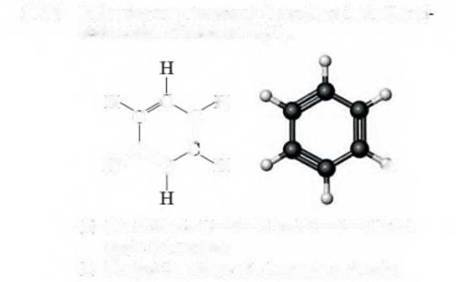# (a) Interpretation: The bond angle should be identified for H-C-C and C-C-C bonds in benzene. Concept Introduction: The bond angles are the angles created in between atoms during the formation of bonds. The bonds angles can be identified with VSEPR model and Lewis structure.BuyFind

### Introduction to General, Organic a...

11th Edition
Frederick A. Bettelheim + 4 others
Publisher: Cengage Learning
ISBN: 9781285869759BuyFind

### Introduction to General, Organic a...

11th Edition
Frederick A. Bettelheim + 4 others
Publisher: Cengage Learning
ISBN: 9781285869759

#### Solutions

Chapter 10, Problem 10.50P
Textbook Problem

##Expert Solution
Interpretation Introduction

(a)

Interpretation:

The bond angle should be identified for H-C-C and C-C-C bonds in benzene.

Concept Introduction:

The bond angles are the angles created in between atoms during the formation of bonds. The bonds angles can be identified with VSEPR model and Lewis structure.

### Explanation of Solution

Lewis structure shows valence electrons on atoms of molecule which are forming bonds with other atoms whereas VSEPR model explains about the bond angles of bond forming atoms. When one atom is surrounded by four region of electron density then it forms tetrahedral planar. Trigonal planar and linear planar are formed when three and two regions of electron density surround an atom respectively. The bond angles of tetrahedral, trigonal and linear planar are 109.50, 1200, 1800 respectively

Expert Solution
Interpretation Introduction

(b)

Interpretation:

The shape of benzene molecule should be identified.

Concept Introduction:

Lewis structure which represents valence electrons of atoms that forms bonds with other atoms is very important to identify shape of molecule. VSEPR model explains about the bond angles of joined atoms also supports Lewis model for understanding structure and shape of molecule.

## Expert Solution

### Want to see the full answer?

Check out a sample textbook solution.

### Want to see this answer and more?

Experts are waiting 24/7 to provide step-by-step solutions in as fast as 30 minutes!*

*Response times may vary by subject and question complexity. Median response time is 34 minutes for paid subscribers and may be longer for promotional offers.© 2021 bartleby. All Rights Reserved.